Home » FAQ » How to Select Variable Frequency Drive Reactor?

# How to Select Variable Frequency Drive Reactor?

Input reactors are used to suppress surge voltage and surge current, protect the variable frequency drive and extend its service life. Since the VFD adopts the frequency conversion method to adjust the speed, high-order harmonics are often generated during the speed regulation to affect the normal use of the equipment. To this end, adding a reactor at the input can improve the power factor of the VFD and suppress harmonic currents, filtering out harmonic voltages and harmonic currents.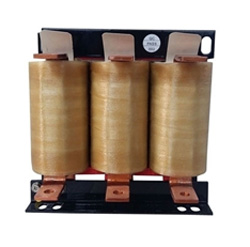Output reactor is used to extend the effective transmission distance of the VFD. It can suppress the instantaneous high voltage generated when the IGBT is switched, reduce the noise and eddy current loss of the motor, and protect the power switching device inside the VFD.

Here are some things about how to choose a reactor.
Selection of Rated AC Current

Rated AC current is the long-term working current for the reactor design from the heating perspective, enough higher harmonic components should be considered at the same time. Namely, the actual current flowing through the output reactor is the output current of the VFD motor load.

Voltage Drop

Voltage drop refers to the actual voltage drop at both ends of reactor coil corresponding to the actual rated current at 50Hz. Generally, the voltage drop is 4V~8V.

Selection of Inductance

Reactor’s rated inductance is also an important parameter! If the inductance is not selected properly, it will directly affect the change of voltage drop under rated current, thus causing faults. The inductance depends on the cross-sectional area of reactor core, the turn number of coil and the adjustment of air gap.
Selection of output reactor inductance is determined according to the length of cable in the rated frequency range. Then, the cross-section areas of iron core and conductor are selected according to the actual rated current of the motor under corresponding inductance requirement, the actual voltage drop can be thereof calculated.

Rated Current, Inductance and Cable length
 Cable Length (meter) Rated Output Current (A) Inductance Value (μH) 300 100 46 200 23 250 16 300 13 600 100 92 200 46 250 34 300 27

For an ideal reactor, its inductance shall remain unchanged at rated AC or below current, its inductance decreases gradually with the current increase.
When the rated current is more than 2 times, the inductance decreases to 0.6 times of the rated inductance.
When the rated current is more than 2.5 times, the inductance decreases to 0.5 times of the rated inductance.
When the rated current is more than 4 times, the inductance decreases to 0.35 times of the rated inductance.

Plz Calculate (9 * 3) =
(Your comment will show after approved.)

You may also like:Variable Frequency Drives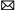info@variablefrequencydrive.net

Featured Articles
Variable Frequency Drive for Fans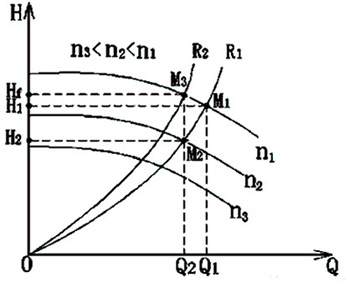Fan is a kind of machine that compresses and conveys gas. If the pressure of exhausted air is quite low, the fan plays a role of ...
Variable Frequency Drive for Food ...Nowadays the food machinery industry has been rapidly developed. As the motor drive, variable frequency drive will change the ...
Can I Run a 60Hz Motor on 50Hz by ...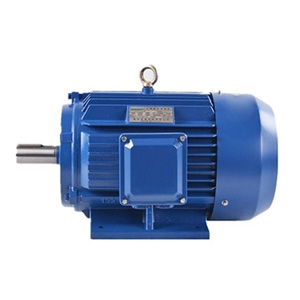We are often asked “can the motor with a rated frequency of 60Hz operate at 50Hz (vice versa)?” When the motor is operated at a ...
How Does Variable Frequency Drive ... The rotating speed of inductor type AC motor (hereinafter referred to as motor) is determined by the number of pole and ...
What is a Variable Frequency Drive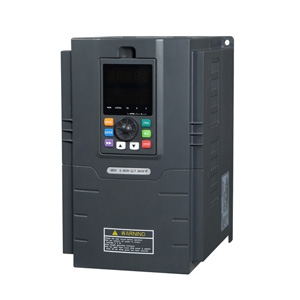Variable frequency drive (VFD) is an adjustable speed drive used for motor drive system, control the speed and torque of the AC ...